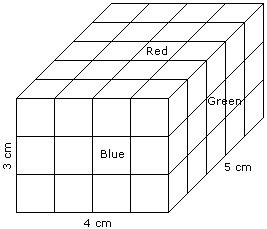# Verbal Reasoning - Cube and Cuboid - Discussion

Discussion Forum : Cube and Cuboid - Cube and Cuboid 3 (Q.No. 2)
Directions to Solve

The following questions are based on the information given below:

1. A cuboid shaped wooden block has 4 cm length, 3 cm breadth and 5 cm height.
2. Two sides measuring 5 cm x 4 cm are coloured in red.
3. Two faces measuring 4 cm x 3 cm are coloured in blue.
4. Two faces measuring 5 cm x 3 cm are coloured in green.
5. Now the block is divided into small cubes of side 1 cm each.

2.
How many small cubes will have only one face coloured ?
12
28
22
16
Explanation:2 from the front + 2 from the back + 3 from the left + 3 from the right + 6 from the top + 6 from the bottom = 22

Discussion:
3 comments Page 1 of 1.

Mohit said:   3 years ago
@Lalit.

Why you subtracting 2?

Lalit ku sahu said:   9 years ago
For cube:

One face coloured= 6*(n-2)^2.
= 6*(n-2)*(n-2).

Similarly for CUBOID (4*3*5):

One face coloured = 2*(L-2)*(B-2)+2*(B-2)*(H-2)+2*(H-2)*(L-2).

=2*(4-2)*(3-2)+2*(3-2)*(5-2)+2*(5-2)*(4-2).

= 2*2*1 + 2*1*3 + 2*3*2.

= 4 + 6 + 12.